Next: Powers modulo a prime Up: Introduction to Number Theory Previous: Congruences   Contents

## The Greatest Common Divisor

For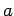and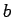, the number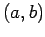is the largest number which dividesandevenly.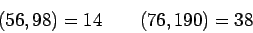Theorem 1   For anythere are integerswith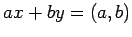Proof: The equation can be solved by making a sequence of simplifying substitutions: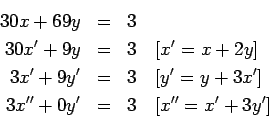It is easy to see that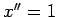,is a solution to the final equation and we get a solution to the original equation by working backwards: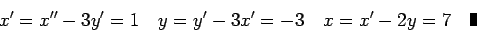We could also solve an equation like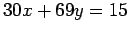by multiplying our solution:,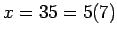. It should be clear that the equation will have no solution in integers if 15 is replaced by something that is not a multiple of.

All other integer solutions ofmay be obtained by changing the first solution: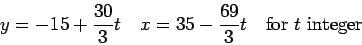If we do the process illustrated on the previous page for any equation, we eventually get one of the coefficients as zero and the other as. [In fact, this process is usually presented as Euclid's algorithm for finding the greatest common divisor.'']

It is important that this process is feasible [on a computer] even ifandare several hundred digits long. It is easy to show that the larger of the two coefficients decreases by at leastevery two equations, hence that in twenty equations the larger coefficient has decreased by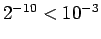, so a 600-digit number would not require more than 4000 equations. [this analysis can be improved]

We pointed out earlier that division does not work with congruences. An important application of Theorem 1 is that it does work for prime numbers.

Corollary 2   If p is a prime number,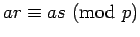, and, then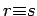.

Proof: Since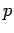is a prime,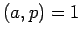, so Theorem 1 says there are integerwith. Hence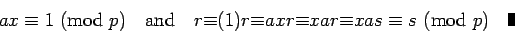Corollary 3   If p is a prime number andmod, then for any, there is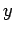with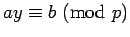.

Proof: We showed in the preceding proof that there is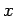with. Let. height 8pt width 4pt

Corollary 4 (The Chinese Remainder Theorem'')   If, then for any, there is an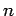with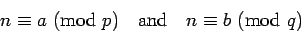Proof: Theorem 1 implies there are integerssuch that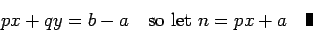Next: Powers modulo a prime Up: Introduction to Number Theory Previous: Congruences   Contents
Translated from LaTeX by Scott Sutherland
2002-12-14